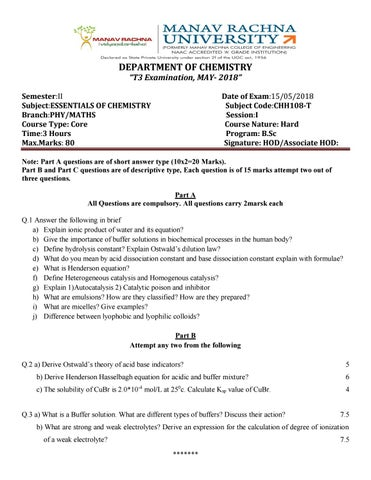DEPARTMENT OF CHEMISTRY “T3 Examination, MAY- 2018” Semester:II Subject:ESSENTIALS OF CHEMISTRY Branch:PHY/MATHS Course Type: Core Time:3 Hours Max.Marks: 80

Date of Exam:15/05/2018 Subject Code:CHH108-T Session:I Course Nature: Hard Program: B.Sc Signature: HOD/Associate HOD:

Note: Part A questions are of short answer type (10x2=20 Marks). Part B and Part C questions are of descriptive type, Each question is of 15 marks attempt two out of three questions. Part A All Questions are compulsory. All questions carry 2marsk each Q.1 Answer the following in brief a) Explain ionic product of water and its equation? b) Give the importance of buffer solutions in biochemical processes in the human body? c) Define hydrolysis constant? Explain Ostwald’s dilution law? d) What do you mean by acid dissociation constant and base dissociation constant explain with formulae? e) What is Henderson equation? f) Define Heterogeneous catalysis and Homogenous catalysis? g) Explain 1)Autocatalysis 2) Catalytic poison and inhibitor h) What are emulsions? How are they classified? How are they prepared? i) What are micelles? Give examples? j) Difference between lyophobic and lyophilic colloids? Part B Attempt any two from the following Q.2 a) Derive Ostwald’s theory of acid base indicators?

5

b) Derive Henderson Hasselbagh equation for acidic and buffer mixture?

6

c) The solubility of CuBr is 2.0*10-4 mol/L at 250c. Calculate Ksp value of CuBr.

4

Q.3 a) What is a Buffer solution. What are different types of buffers? Discuss their action?

7.5

b) What are strong and weak electrolytes? Derive an expression for the calculation of degree of ionization of a weak electrolyte?

7.5 *******

Q.4 Discuss the following theories with an example each 1) Bronsted â&#x20AC;&#x201C;Lowry concept 2) Lewis concept of acids and bases 3)Arhenius concept of acid and bases

(3*5=15)

Part C Attempt any two from the following Q.5 a)What is catalysis? Derive the Michaelis-Menten equation to explain enzyme catalysis 7.5 b) Write short notes on BET equation, and explain its terms? 7.5 Q.6 a) What are the differences between physical adsorption and chemical adsorption? 7.5 b) Write short notes on Intermediate compound formation theory? 7.5 Q.7 a) What are the characteristic feature of enzyme catalysis? 7.5 b) Write short notes on adsorption theory and explain how it effects on hydrogenation of ethane in presence of nickel. 5 c) Write short notes on acid base catalysis? 2.5

*******